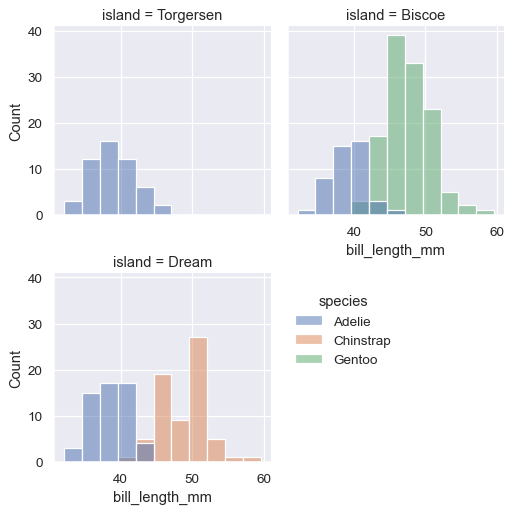# seaborn.move_legend#

seaborn.move_legend(obj, loc, **kwargs)#

Recreate a plot’s legend at a new location.

The name is a slight misnomer. Matplotlib legends do not expose public control over their position parameters. So this function creates a new legend, copying over the data from the original object, which is then removed.

Parameters:
objthe object with the plot

This argument can be either a seaborn or matplotlib object:

locstr or int

Location argument, as in `matplotlib.axes.Axes.legend()`.

kwargs

Other keyword arguments are passed to `matplotlib.axes.Axes.legend()`.

Examples

For axes-level functions, pass the `matplotlib.axes.Axes` object and provide a new location.

```ax = sns.histplot(penguins, x="bill_length_mm", hue="species")
sns.move_legend(ax, "center right")
```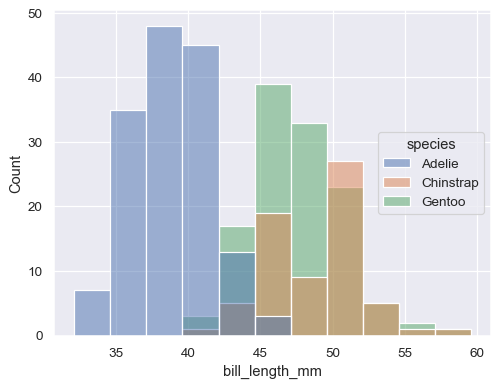Use the `bbox_to_anchor` parameter for more fine-grained control, including moving the legend outside of the axes:

```ax = sns.histplot(penguins, x="bill_length_mm", hue="species")
sns.move_legend(ax, "upper left", bbox_to_anchor=(1, 1))
```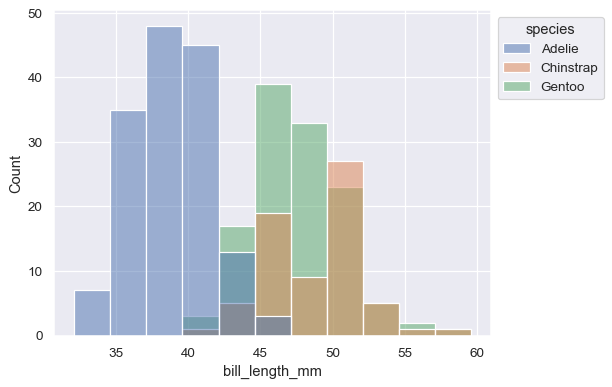Pass additional `matplotlib.axes.Axes.legend()` parameters to update other properties:

```ax = sns.histplot(penguins, x="bill_length_mm", hue="species")
sns.move_legend(
ax, "lower center",
bbox_to_anchor=(.5, 1), ncol=3, title=None, frameon=False,
)
```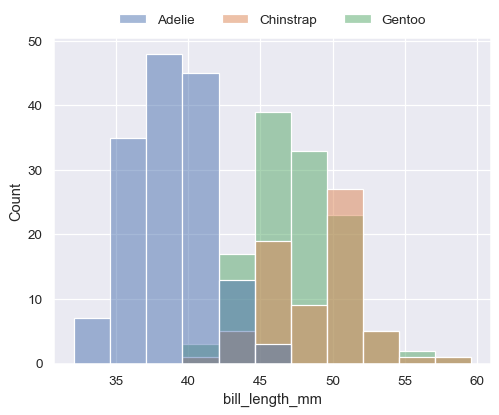It’s also possible to move the legend created by a figure-level function. But when fine-tuning the position, you must bear in mind that the figure will have extra blank space on the right:

```g = sns.displot(
penguins,
x="bill_length_mm", hue="species",
col="island", col_wrap=2, height=3,
)
sns.move_legend(g, "upper left", bbox_to_anchor=(.55, .45))
```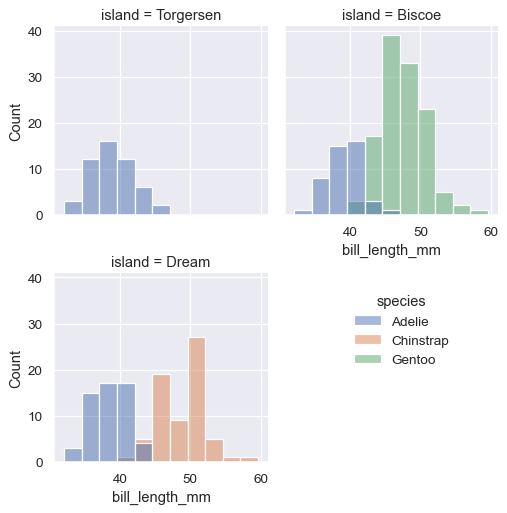One way to avoid this would be to set `legend_out=False` on the `FacetGrid`:

```g = sns.displot(
penguins,
x="bill_length_mm", hue="species",
col="island", col_wrap=2, height=3,
facet_kws=dict(legend_out=False),
)
sns.move_legend(g, "upper left", bbox_to_anchor=(.55, .45), frameon=False)
```